# Revision Index Page 2 Page 9 Page 16

• Slides: 38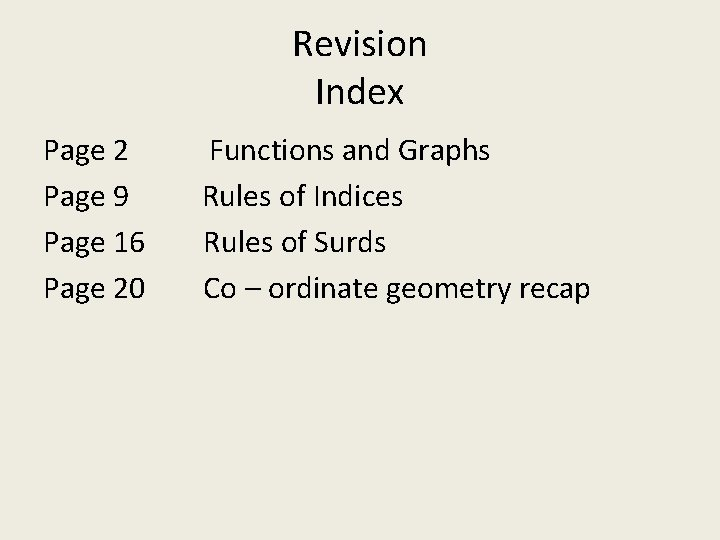Revision Index Page 2 Page 9 Page 16 Page 20 Functions and Graphs Rules of Indices Rules of Surds Co – ordinate geometry recap2009 Q 5 (a) “f(x) = 5 x – 12 and =a ” f(a) = 5 a – 12 a = 5 a – 12 4 a = 12 a=3 f(a)(b)(i) f(x) = 5 x – 4 g(x) = 3 x + 1 Domain = 0 ≤ x ≤ 3 x = 0, 1, 2, 3 f(1) = 5(0) – 4 = -4 (0, -4) f(3) = 5(3) – 4 = 11 (3, 11) g(1) = 3(0) + 1 = 1 (0, 1) g(3) = 3(3) + 1 = 10 (3, 10) (ii) P. O. I = (2. 5, 8. 5)(-4, 13) (-3, 0) (-3, -9) (-1, 14) (0, -15) (1, -12) (2, -5) (3, 6) (ii) Minimum value of f(x) (iii)Range of values of x for which f(x) ≥ 0Q 5 2008 P 1 (i) Fnd the value of b and c (ii) Solve f(x) = -6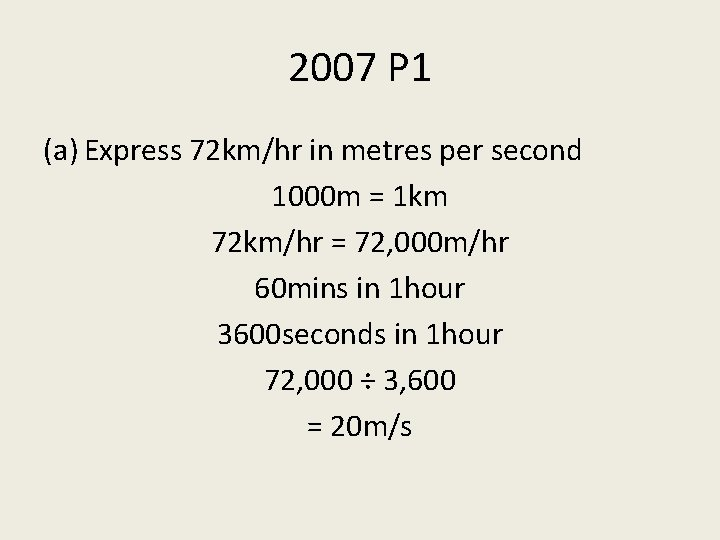2007 P 1 (a) Express 72 km/hr in metres per second 1000 m = 1 km 72 km/hr = 72, 000 m/hr 60 mins in 1 hour 3600 seconds in 1 hour 72, 000 ÷ 3, 600 = 20 m/s(ii) Snowman with mass of 12 kg Melts at a rate of 0. 2% of its mass per minute What is its mass after 3 mins Mass at start of 1 st min: Less 0. 2% Mass after 1 min 12 kg 0. 024 kg 11. 976 kg Mass at start of 2 nd min: Less 0. 2% Mass after 2 mins 11. 976 0. 23952 kg 11. 952 kg Mass at start of 3 rd min: Less 0. 2% Mass after 3 mins 11. 952 0. 23904 kg 11. 928 kg Mass after 3 min = 11. 93 kg(c) Indices Rules of Indices an x a m = a n + m an/am = an – m Rule 2: (4³)² = 4⁶ Example: 4⁶ x 4³ = 4⁹ 5⁷ 5⁵ = 5² Example: Express 9⁴ in the form 3 n 9 = 3² 9⁴ = (3²)⁴ 9⁴ = 3⁸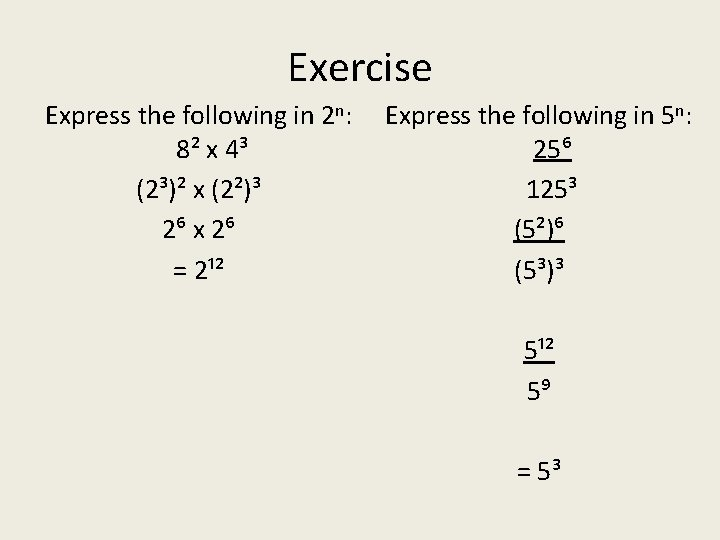Exercise Express the following in 2 n: 8² x 4³ (2³)² x (2²)³ 2⁶ x 2⁶ = 2¹² Express the following in 5 n: 25⁶ 125³ (5²)⁶ (5³)³ 5¹² 5⁹ = 5³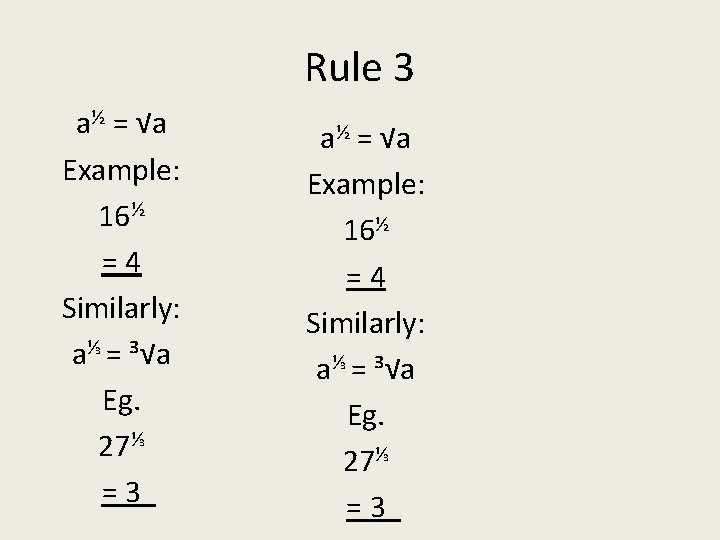Rule 3 a½ = √a Example: 16½ =4 Similarly: a⅓ = ³√a Eg. 27⅓ =32006 3(a) paper 1 (i) (64)³⁄₂ (64½)³ 8³ = 512 (ii) (64)⅔ (64¹⁄₃)² 4² = 16 2005 3(a) paper 1 in the form 2 k = 16⅓ = (2³)⅓ = 2³⁄₃ = 2¹ k=1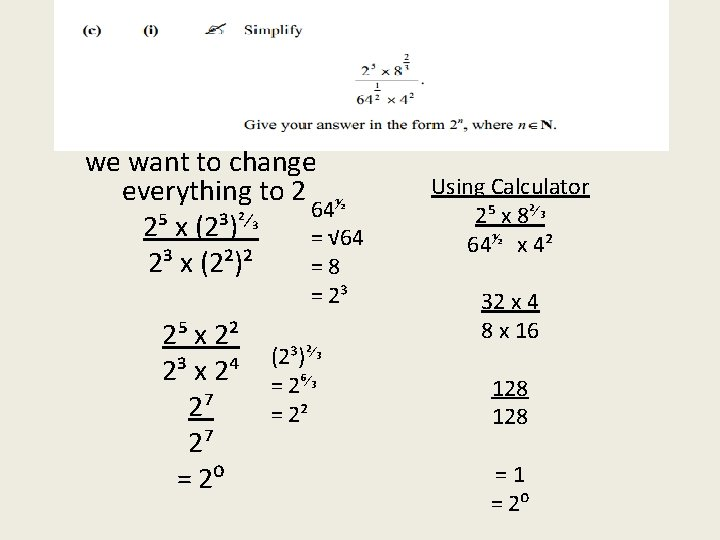we want to change everything to 2 ½ 64 ²⁄₃ 2⁵ x (2³) = √ 64 2³ x (2²)² =8 = 2³ 2⁵ x 2² 2³ x 2⁴ 2⁷ 2⁷ = 2⁰ (2³)²⁄₃ = 2⁶⁄₃ = 2² Using Calculator 2⁵ x 8²⁄₃ 64½ x 4² 32 x 4 8 x 16 128 =1 = 2⁰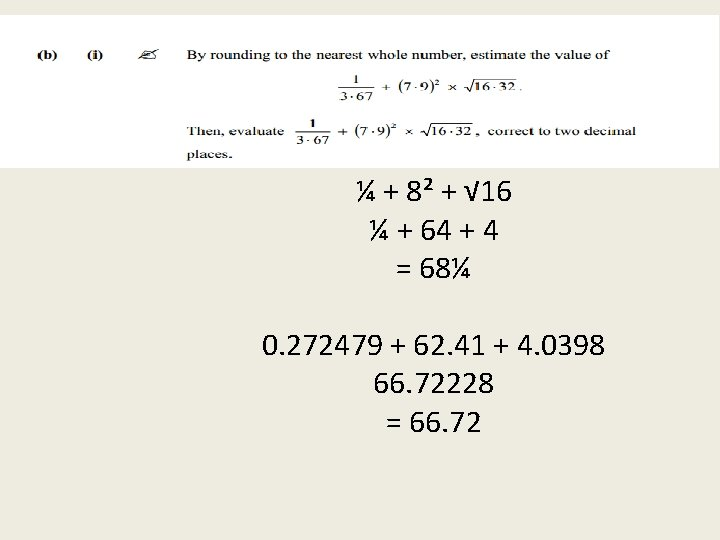2003 Q 2(b) ¼ + 8² + √ 16 ¼ + 64 + 4 = 68¼ 0. 272479 + 62. 41 + 4. 0398 66. 72228 = 66. 72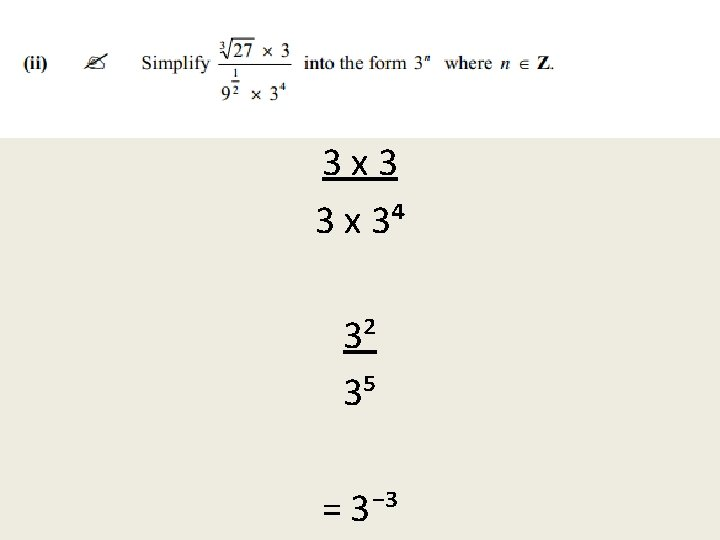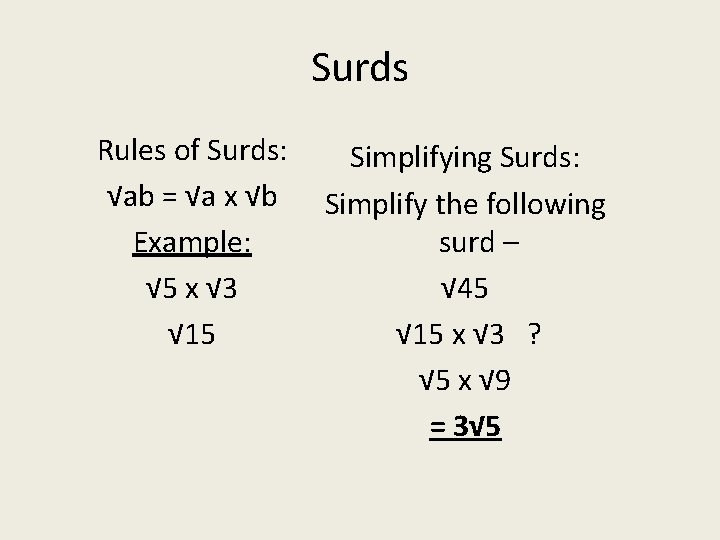Surds Rules of Surds: √ab = √a x √b Example: √ 5 x √ 3 √ 15 Simplifying Surds: Simplify the following surd – √ 45 √ 15 x √ 3 ? √ 5 x √ 9 = 3√ 5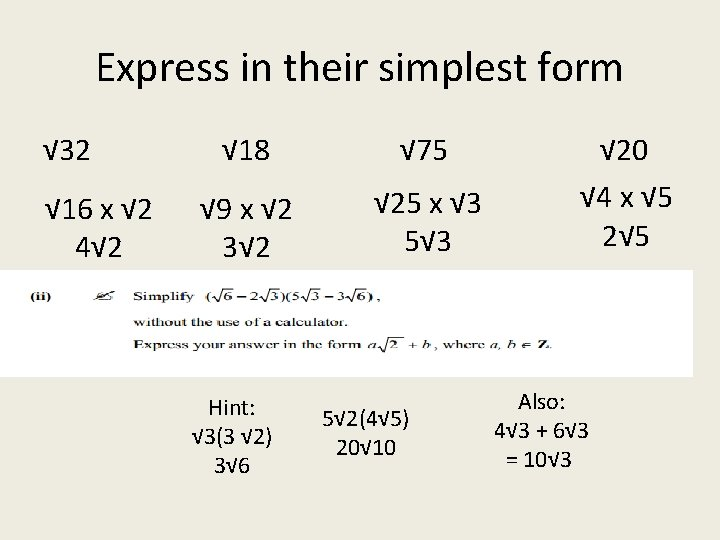Express in their simplest form √ 32 √ 16 x √ 2 4√ 2 √ 18 √ 75 √ 9 x √ 2 3√ 2 √ 25 x √ 3 5√ 3 Hint: √ 3(3 √ 2) 3√ 6 5√ 2(4√ 5) 20√ 10 √ 20 √ 4 x √ 5 2√ 5 Also: 4√ 3 + 6√ 3 = 10√ 3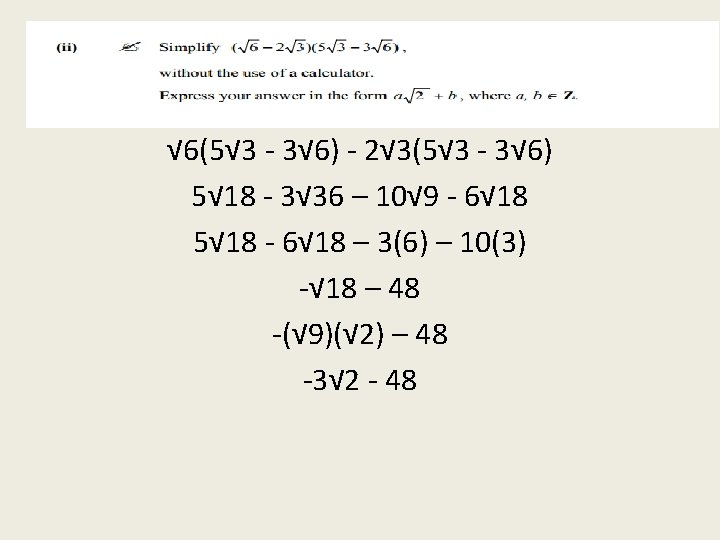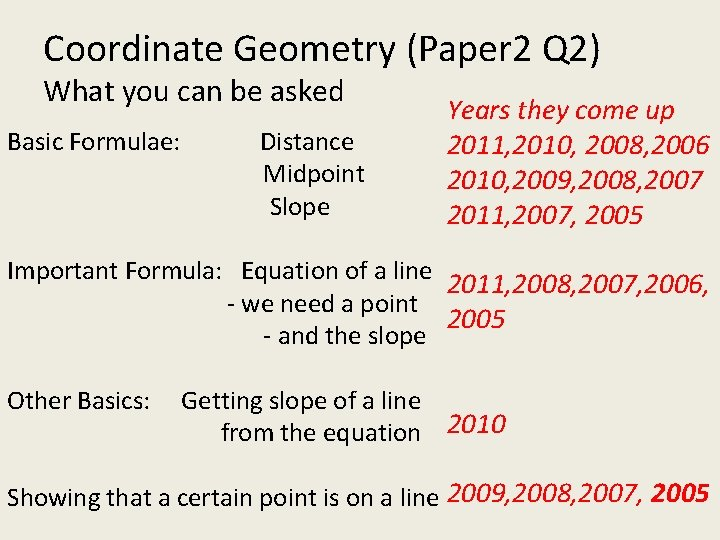Coordinate Geometry (Paper 2 Q 2) What you can be asked Basic Formulae: Distance Midpoint Slope Years they come up 2011, 2010, 2008, 2006 2010, 2009, 2008, 2007 2011, 2007, 2005 Important Formula: Equation of a line 2011, 2008, 2007, 2006, - we need a point 2005 - and the slope Other Basics: Getting slope of a line from the equation 2010 Showing that a certain point is on a line 2009, 2008, 2007, 2005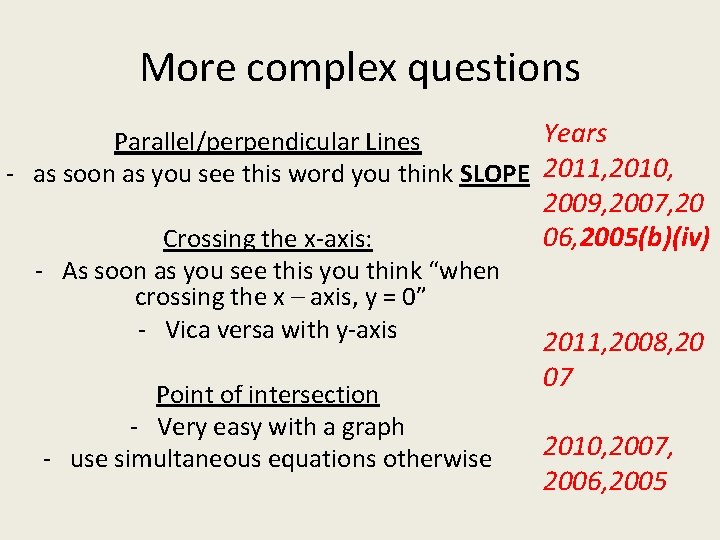More complex questions Years Parallel/perpendicular Lines - as soon as you see this word you think SLOPE 2011, 2010, Crossing the x-axis: - As soon as you see this you think “when crossing the x – axis, y = 0” - Vica versa with y-axis Point of intersection - Very easy with a graph - use simultaneous equations otherwise 2009, 2007, 20 06, 2005(b)(iv) 2011, 2008, 20 07 2010, 2007, 2006, 2005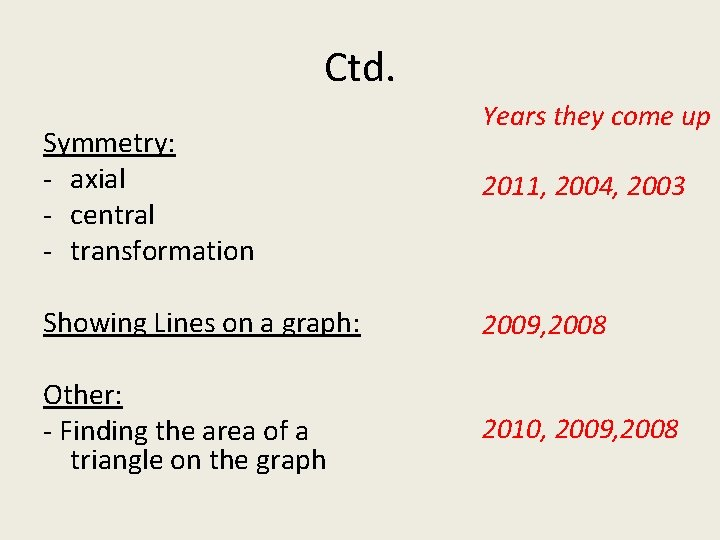Ctd. Symmetry: - axial - central - transformation Years they come up 2011, 2004, 2003 Showing Lines on a graph: 2009, 2008 Other: - Finding the area of a triangle on the graph 2010, 2009, 2008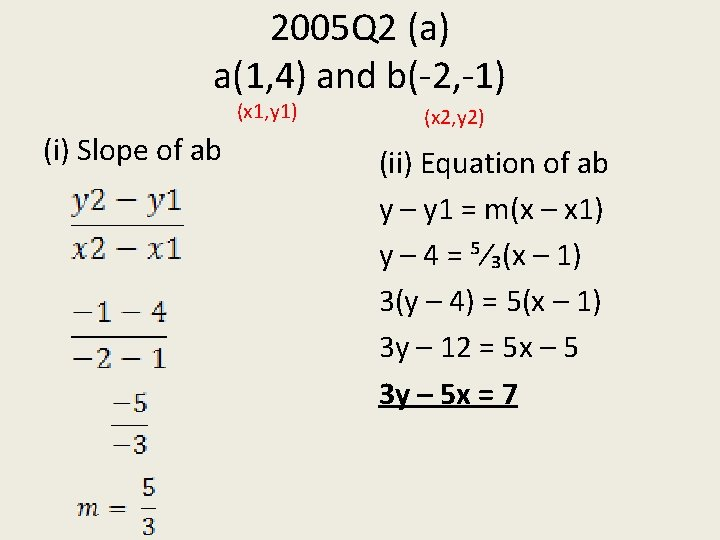2005 Q 2 (a) a(1, 4) and b(-2, -1) (x 1, y 1) (i) Slope of ab (x 2, y 2) (ii) Equation of ab y – y 1 = m(x – x 1) y – 4 = ⁵⁄₃(x – 1) 3(y – 4) = 5(x – 1) 3 y – 12 = 5 x – 5 3 y – 5 x = 7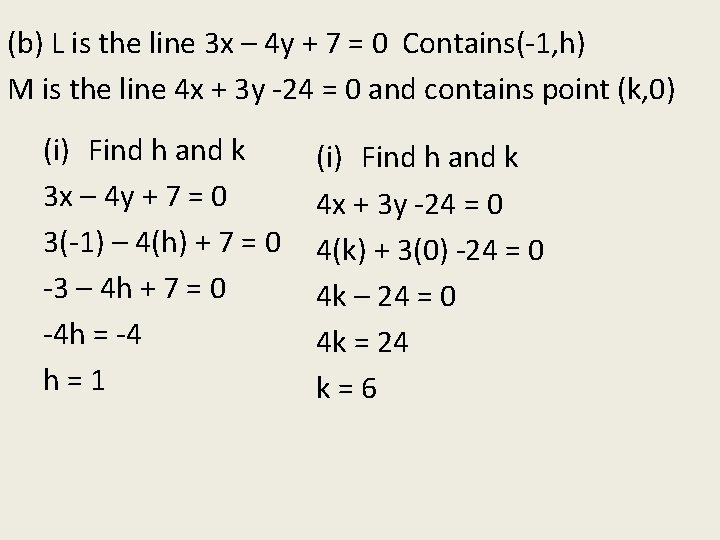(b) L is the line 3 x – 4 y + 7 = 0 Contains(-1, h) M is the line 4 x + 3 y -24 = 0 and contains point (k, 0) (i) Find h and k 3 x – 4 y + 7 = 0 3(-1) – 4(h) + 7 = 0 -3 – 4 h + 7 = 0 -4 h = -4 h=1 (i) Find h and k 4 x + 3 y -24 = 0 4(k) + 3(0) -24 = 0 4 k – 24 = 0 4 k = 24 k=6(b) L is the line 3 x – 4 y + 7 = 0 Contains(-1, h) M is the line 4 x + 3 y -24 = 0 and contains point (k, 0) (ii) Point of intersection of L and M is r 3 x – 4 y = -7 x=3 4 x + 3 y = 24 3 x – 4 y = -7 3(3) – 4 y = -7 9 x – 12 y = -21 16 x + 12 y = 96 y = 4 25 x = 75 P. O. I = (3, 4) x=3On a graph p(-1, 1) q(6, 0) r(4, 3)Prove it’s a Right Angle If it’s a right angle – the lines are perpendicular i. e. their slopes multiplied give you -1 Slope of L: 3 x – 4 y + 7 = 0 -b/a = -(-4)/3 m₁= ⁴⁄₃ Slope of M: 4 x + 3 y – 24 = 0 -b/a = -3/4 = m₂ = -¾ ⁴⁄₃ x (-¾) = -1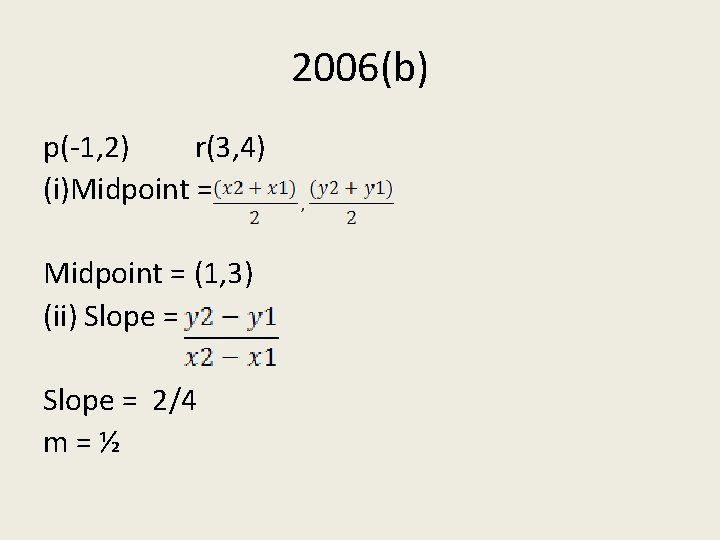2006(b) p(-1, 2) r(3, 4) (i)Midpoint = (1, 3) (ii) Slope = 2/4 m=½(iii) Find equation of perpendicular bisector How will we find the bisector? Midpoint! Wait! We already have it! Midpoint = (1, 3) A perpendicular line Slope is inverse Slope of pr = ½ Slope of L = -2/1 or Slope = -2 Equation = y – y 1 =m(x – x 1) y – 3 = -2(x – 1) y + 2 x = 10(iv) Equation of K is x – 2 y = 0 Point of intersection L: y + 2 x = 5 K: -2 y + x = 0 2 y + 4 x = 10 -2 y + x = 0 5 x = 10 x=2 y + 2 x = 5 y+8=5 y=1 Point of Intersection= (2, 1)Trigonometry 2006 Paper 2 Q 5 (a) Construct the angle A such that Tan A = ¾ Tan = opposite/adjacent We construct a right angled triangle where the opposite side is 3 and the adjacent is 4 3 A 4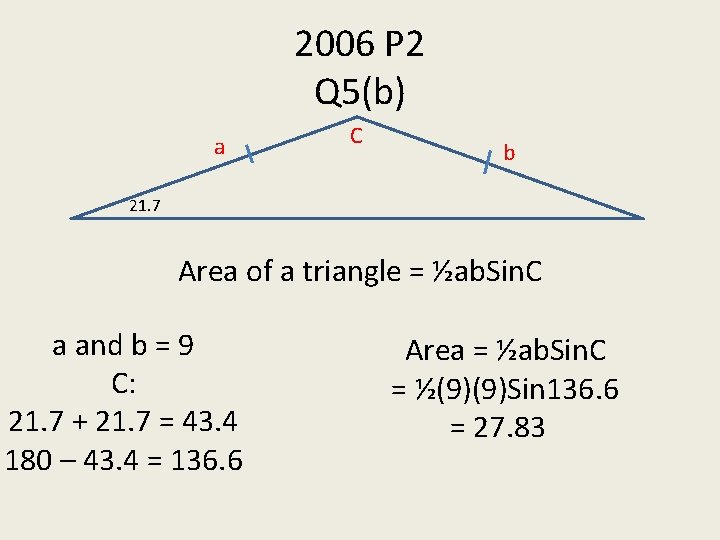2006 P 2 Q 5(b) a C b 21. 7 Area of a triangle = ½ab. Sin. C a and b = 9 C: 21. 7 + 21. 7 = 43. 4 180 – 43. 4 = 136. 6 Area = ½ab. Sin. C = ½(9)(9)Sin 136. 6 = 27. 83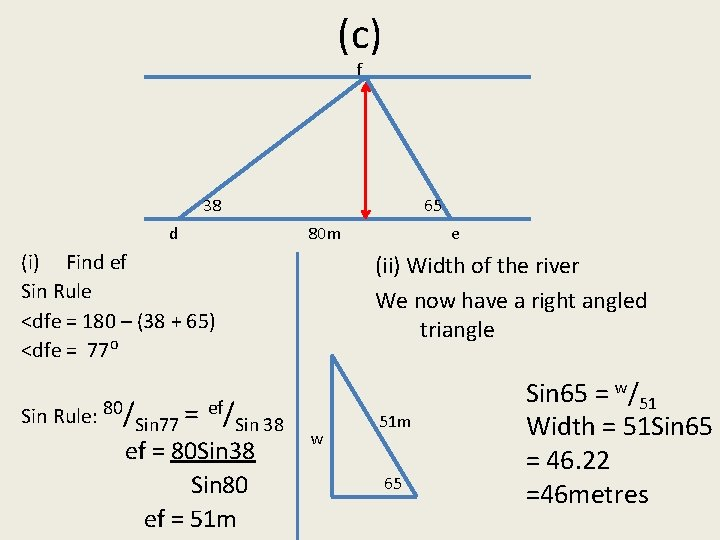(c) f 38 d 65 80 m (i) Find ef Sin Rule <dfe = 180 – (38 + 65) <dfe = 77⁰ Sin Rule: 80/Sin 77 = ef/Sin 38 ef = 80 Sin 38 Sin 80 ef = 51 m e (ii) Width of the river We now have a right angled triangle w 51 m 65 Sin 65 = w/51 Width = 51 Sin 65 = 46. 22 =46 metres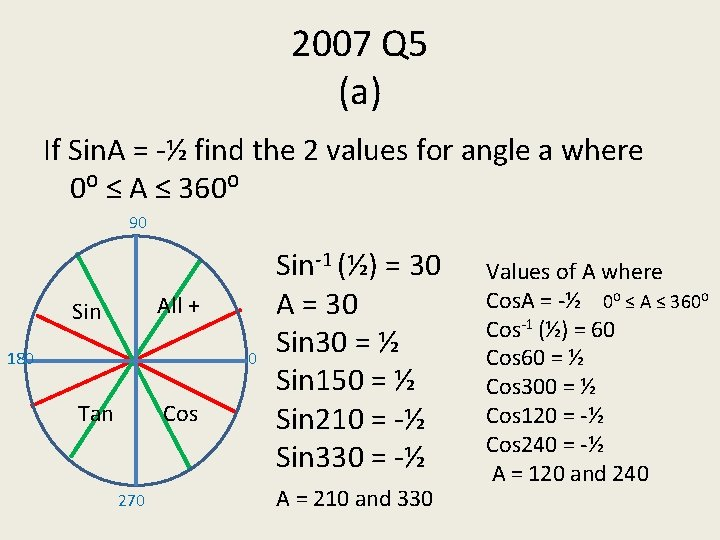2007 Q 5 (a) If Sin. A = -½ find the 2 values for angle a where 0⁰ ≤ A ≤ 360⁰ 90 All + Sin 180 0 Tan Cos 270 Sin-1 (½) = 30 A = 30 Sin 30 = ½ Sin 150 = ½ Sin 210 = -½ Sin 330 = -½ A = 210 and 330 Values of A where Cos. A = -½ 0⁰ ≤ A ≤ 360⁰ Cos-1 (½) = 60 Cos 60 = ½ Cos 300 = ½ Cos 120 = -½ Cos 240 = -½ A = 120 and 240Q 5(b) a 9 km 15 km d c (ii) Find CD Tan 36. 87 = 15/CD CD = 15/Tan 36. 87 CD = 20 km ac = 15 12 km 36. 87 (i) Find ac Pythagoras: 9² + 12² = ac² = 225 (ii) Find AD 20² + 15² = AD² AD = 25 Total Course = 25 + 9 + 12 + 20 = 66 km(c) 10 h x w 30 150 12 m 20 For the Sine rule we need 2 angles and a side 180 – 30 = 150 180 –(150 + 20) = 10 Sine rule: 12 = x Sin 10 Sin 20 x = 12 Sin 20 Sin 10 x = 23. 64(c) (ii) Find h Sin 30 = h/23. 64 10 h 11. 81 h = 23. 64 Sin 30 h = 11. 81 m 23. 64 (iii) Find w w 30 150 12 m 20 Tan 30 = 11. 81/w w = 11. 81/Tan 30 w = 20. 45 m